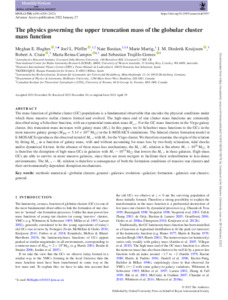# The physics governing the upper truncation mass of the globular cluster mass function

Hughes, ME, Pfeffer, JL, Bastian, N, Martig, M, Kruijssen, JMD, Crain, RA, Reina-Campos, M and Trujillo-Gomez, S (2022) The physics governing the upper truncation mass of the globular cluster mass function. Monthly Notices of the Royal Astronomical Society, 510 (4). pp. 6190-6200. ISSN 0035-8711Preview
Text
The physics governing the upper truncation mass of the globular cluster.pdf - Published Version

The mass function of globular cluster (GC) populations is a fundamental observable that encodes the physical conditions under which these massive stellar clusters formed and evolved. The high-mass end of star cluster mass functions are commonly described using a Schechter function, with an exponential truncation mass $M_{c,*}$. For the GC mass functions in the Virgo galaxy cluster, this truncation mass increases with galaxy mass ($M_{*}$). In this paper we fit Schechter mass functions to the GCs in the most massive galaxy group ($M_{\mathrm{200}} = 5.14 \times 10^{13} M_{\odot}$) in the E-MOSAICS simulations. The fiducial cluster formation model in E-MOSAICS reproduces the observed trend of $M_{c,*}$ with $M_{*}$ for the Virgo cluster. We therefore examine the origin of the relation by fitting $M_{c,*}$ as a function of galaxy mass, with and without accounting for mass loss by two-body relaxation, tidal shocks and/or dynamical friction. In the absence of these mass-loss mechanisms, the $M_{c,*}$-$M_{*}$ relation is flat above $M_* > 10^{10} M_{\odot}$. It is therefore the disruption of high-mass GCs in galaxies with $M_{*}\sim 10^{10} M_{\odot}$ that lowers the $M_{c,*}$ in these galaxies. High-mass GCs are able to survive in more massive galaxies, since there are more mergers to facilitate their redistribution to less-dense environments. The $M_{c,*}-M_*$ relation is therefore a consequence of both the formation conditions of massive star clusters and their environmentally-dependent disruption mechanisms.View Item